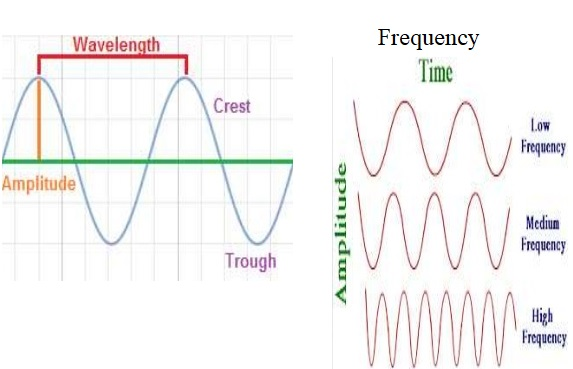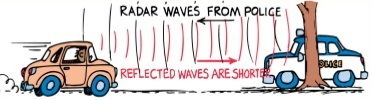# What is Doppler Effect Definition Equation and Formula? [Answered]

Definition of Doppler Effect

Doppler effect is the change in frequency of a wave for an observer moving relative to its source. The observer observes an upward shift in frequency when the wave source is approaching, and a downward shift in frequency when the wave source is retreating. In other words, the doppler effect refers to the change in pitch of a sound due to the motion either of the source or of the listener.

First explained in 1842 by Christian Doppler, the Doppler Effect is the shift in frequency and wavelength of waves which results from a source moving with respect to the medium, a receiver moving with respect to the medium, or even a moving medium.

## Doppler Effect Characteristics

If two objects are approaching each other, or if an initial object is approaching a second standing object, the pitch is higher. If two objects are moving apart, or if an initial object is moving apart from a second standing object, the pitch is lower. The Doppler effect causes the changing pitch of a siren. When a firetruck approaches, the pitch sounds higher than normal because the sound wave crests arrive more frequently. When the firetruck passes and moves away, you hear a drop in pitch because the wave crests are arriving less frequently. This is also worth noting that the change in loudness is not the Doppler Effect! It is the shift in frequency!In general doppler effect applies to all waves including sound waves, light waves and water waves.

### Doppler Effect Equation

To understand the concept of doppler effect we must first understand wavelength, frequency and velocity.

Wavelength is the distance between two successive crest of a wave, especially points in a sound wave or electromagnetic wave.

𝝀 = 𝝊 𝒇 where 𝜆 is wavelength. 𝜐 is speed and 𝑓 is frequency.

Frequency is the number of occurrence of repeating event per unit time. In terms of waves, it’s the number of waves passing a given point per unit time. Unit of frequency is hertz 𝒇 = 𝝂 𝝀.

Velocity is the speed of something (waves) in a given direction.

𝐯 = 𝐝 𝐭 where v is velocity, d is displacement and t is time.The perceived frequency (f ´) is related to the actual frequency (fo) and the relative speeds of the source(vs), the speed (v) of waves in the medium by an equation:

f2 = fo ( v/ v+ v= fo ( v/ v+ vs)) if the source is moving away from the observer

f2 = fo ( v/ v- v= fo ( v/ v- vs)) if the source is moving toward the observer

The choice of using the plus (+) or minus (-) sign is made according to the convention that if the source and observer are moving towards each other the perceived frequency (f ´) is higher than the actual frequency (fo).

Likewise, if the source and observer are moving away from each other the perceived frequency (f ´) lower than the actual frequency (fo).

#### The general Doppler effect Formula

𝒇′ = ((𝝂 + 𝝂s) / (𝝂 − 𝝂o))𝒇

This equation applies to all four conditions mention previously with respect to movements of observer and source. The sign of 𝜈s and 𝜈depend on the direction of the velocity. A positive value is used for motion of the observer or the source toward the other, and a negative value is used for motion of one away from the other.

##### Application and Examples of Doppler Effect

One application of Doppler effect found in nature, occurs in bats hunting for their prey. Bats navigates it’s flight by emitting whistles and listening for the echoes. When chasing prey, its brain detects a change in pitch between the emitted whistle, and the echo it receives. This tells the bat the speed of its target, and the bat adjusts its own speed accordingly.

The Doppler effect is used in some types of radar, to measure the speed of detected objects. For example a police officer uses radar guns to check for speeding vehicles. The radar gun emits waves at a particular frequency, which when strikes the vehicles bounce back toward the gun. The radar gun then measure the frequency of the returning waves, then eventually determine the speed.Doppler radar (radar in general) is a form of technology used not only by law-enforcement officers, but also by meteorologists. Meteorologists utilize Doppler effect to determine the direction and velocity of raindrops, wind direction and other weather events. This principle is very crucial as it allows meteorologist to predict weather pattern including coming storm etc.

Medical diagnosis: Physicians and medical technicians apply Doppler effect to measure the rate and direction of blood flow in a patient’s body, along with ultra-sound. Ultrasound beam are pointed towards an artery, and the reflected waves exhibit a shift in frequency, because the blood cells are acting as moving sources of sound waves. An echocardiogram uses sound waves transmitted by ultrasound to produce images of the heart (transducer transmit and receive waves, which are reflected when they reach the edge of two structures with different densities).

Flow Measurement: Instruments such as the laser Doppler velocimeter (LDV), and Acoustic Doppler Velocimeter (ADV) have been developed to measure velocities in a fluid flow. A light beam or an ultrasonic acoustic burst is release by the LDV and ADV respectively, subsequently measuring the shift in wavelengths of reflections from particles moving with the flow. This technique allows non-intrusive flow measurements, at high precision and high frequency.

Satellite Communication: Satellite employs doppler effect in its tracking technique for determining distance between satellite and receiver at the time of closest approach as well as the time itself. Approaching satellite increases the frequency relative to the actual transmission frequency. As it retreats, the frequency lowered. At the time of closest approach, the transmitted and received frequencies are usually the same.

Doppler effect has found its use in several other area which includes; Astronomy, Vibration measurement, To Sense Gesture (computer base), Audio and Velocity profile measurement etc.

error: Content is protected !!